# Atomic Structure Questions and Answers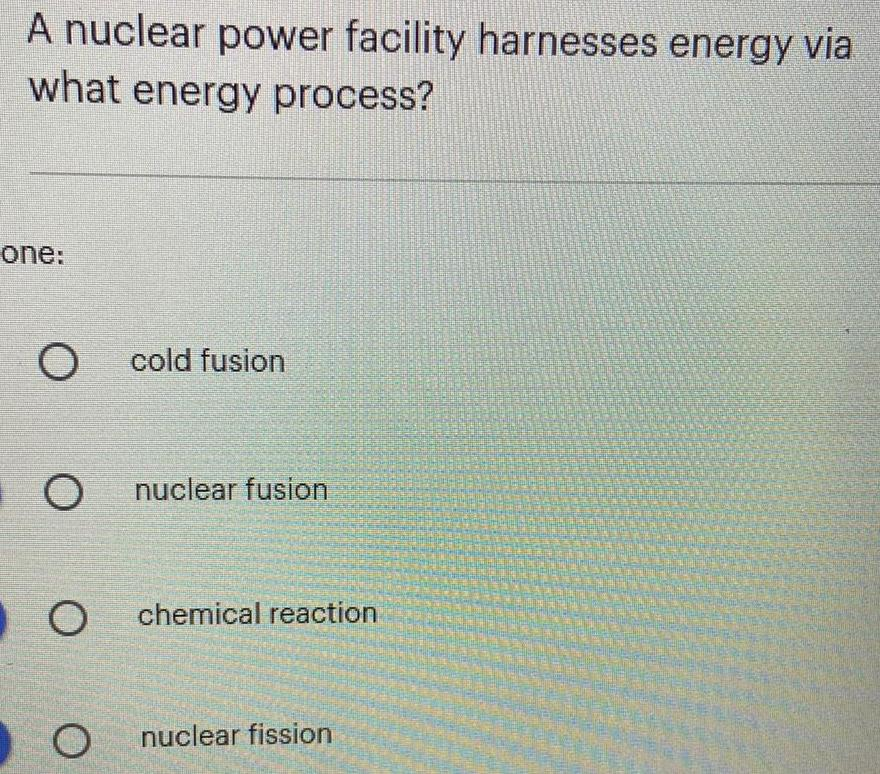Physics
Atomic Structure
A nuclear power facility harnesses energy via what energy process? O cold fusion O nuclear fusion O chemical reaction O nuclear fission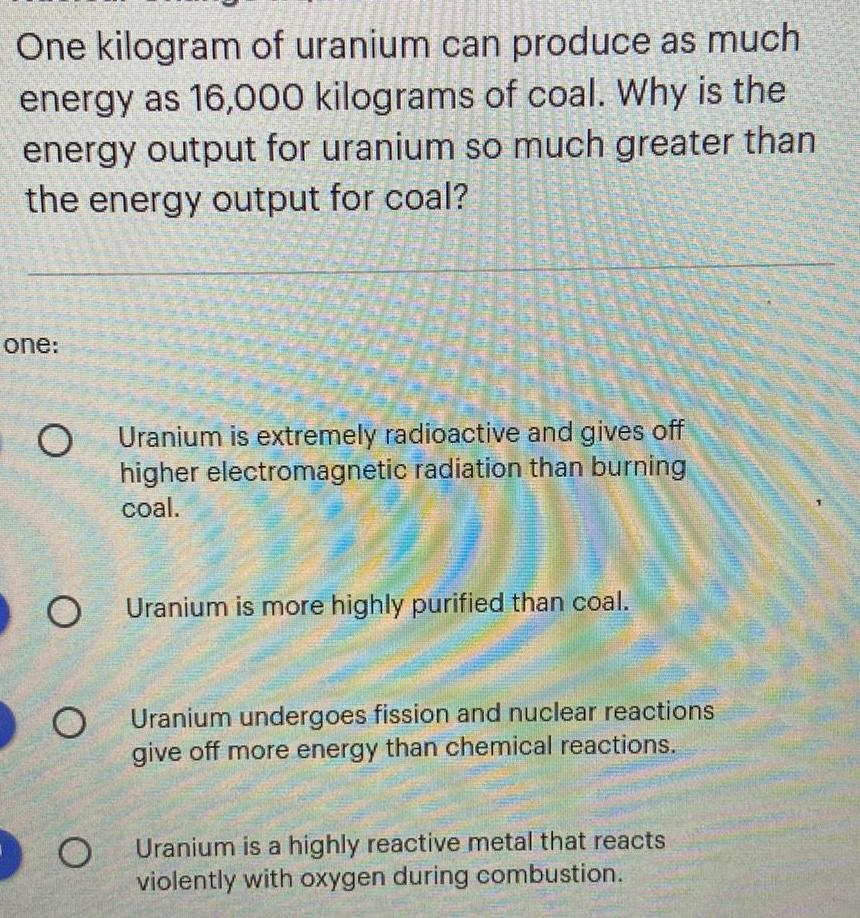Physics
Atomic Structure
One kilogram of uranium can produce as much energy as 16,000 kilograms of coal. Why is the energy output for uranium so much greater than the energy output for coal? A. Uranium is more highly purified than coal. B. Uranium is extremely radioactive and gives off higher electromagnetic radiation than burning coal. C. Uranium undergoes fission and nuclear reactions give off more energy than chemical reactions. D. Uranium is a highly reactive metal that reacts violently with oxygen during combustion.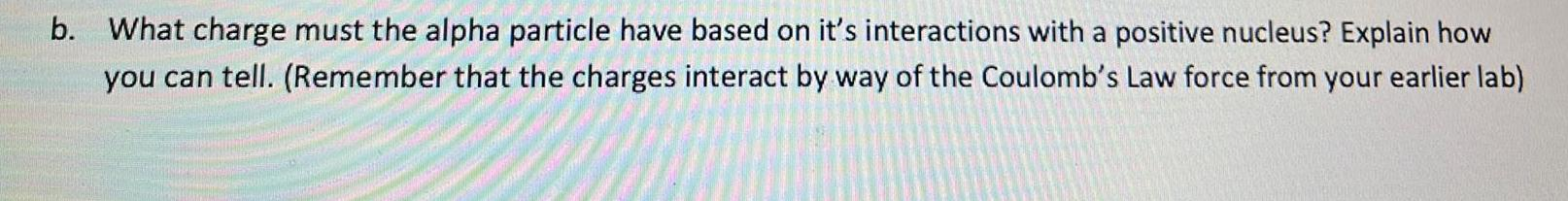Physics
Atomic Structure
What charge must the alpha particle have based on it's interactions with a positive nucleus? Explain how you can tell. (Remember that the charges interact by way of the Coulomb's Law force from your earlier lab)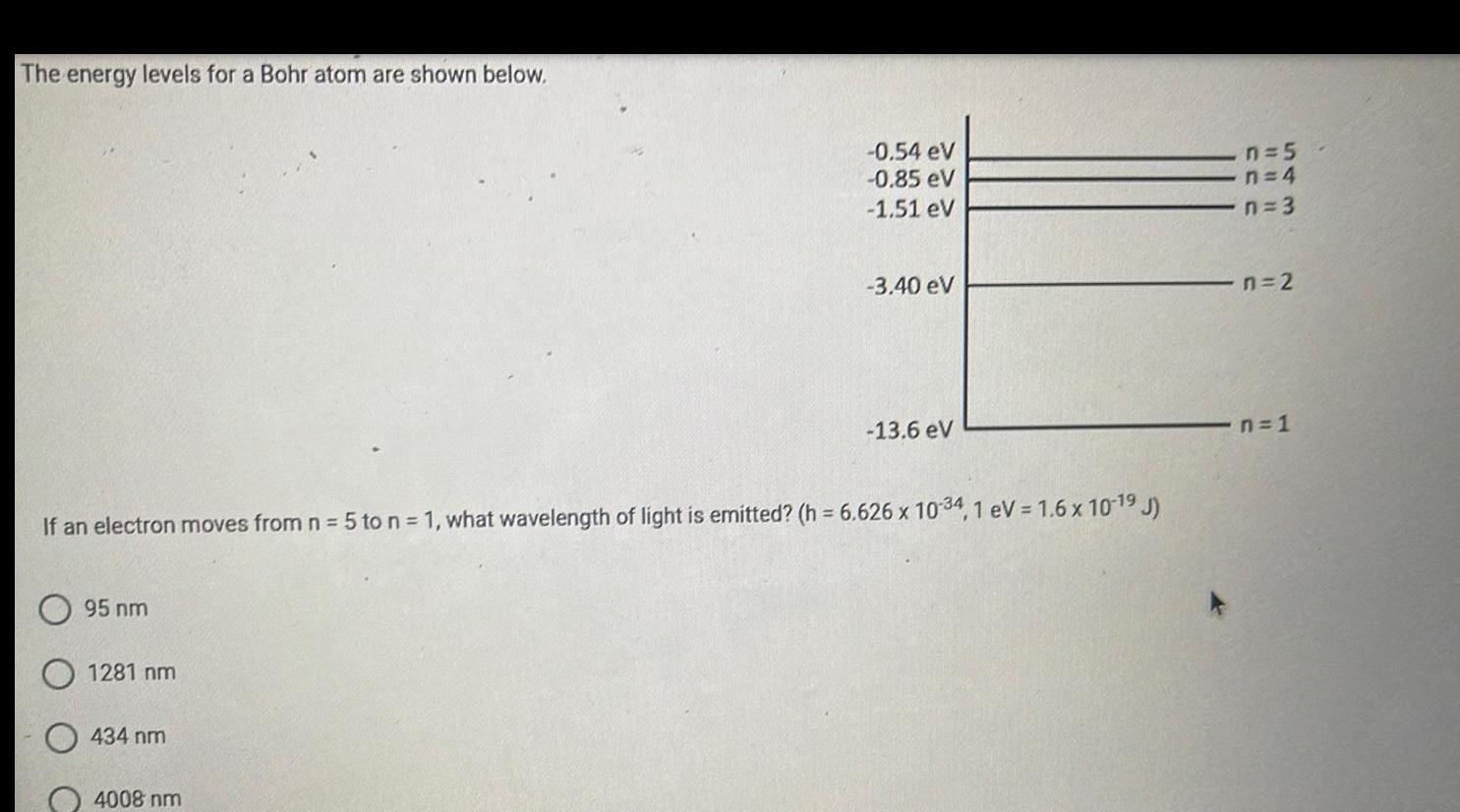Physics
Atomic Structure
The energy levels for a Bohr atom are shown below. If an electron moves from n = 5 to n = 1, what wavelength of light is emitted? (h= 6.626 x 10-34, 1 eV = 1.6 x 10-¹⁹ J) 95 nm 1281 nm 434 nm 4008 nm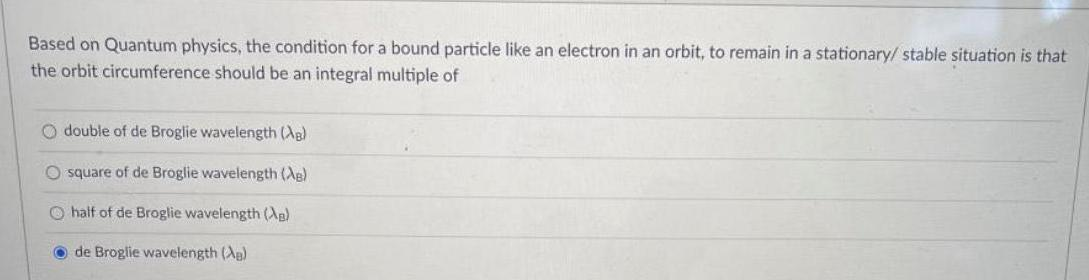Physics
Atomic Structure
Based on Quantum physics, the condition for a bound particle like an electron in an orbit, to remain in a stationary/ stable situation is that the orbit circumference should be an integral multiple of double of de Broglie wavelength (AB) square of de Broglie wavelength (Ag) half of de Broglie wavelength (AB) de Broglie wavelength (AB)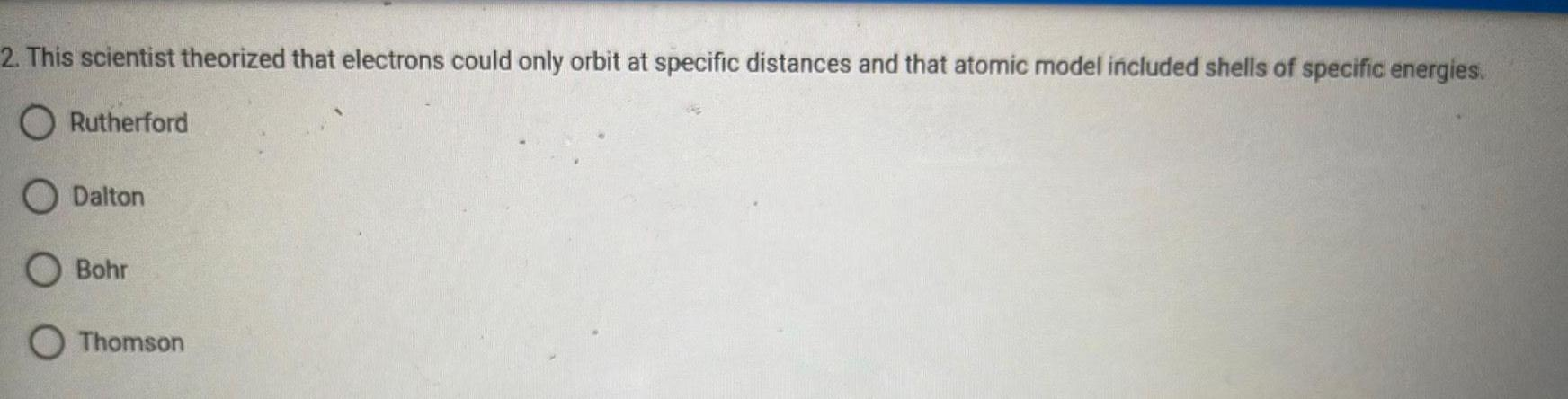Physics
Atomic Structure
2. This scientist theorized that electrons could only orbit at specific distances and that atomic model included shells of specific energies. Rutherford Dalton Bohr Thomson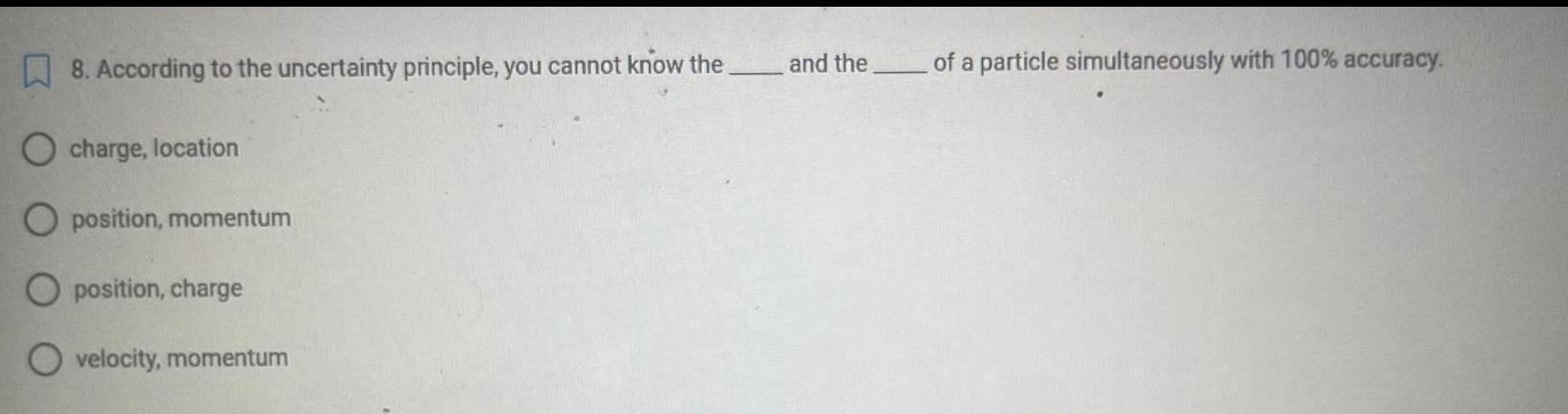Physics
Atomic Structure
8. According to the uncertainty principle, you cannot know the ___ and the ___ of a particle simultaneously with 100% accuracy. charge, location position, momentum position, charge velocity, momentum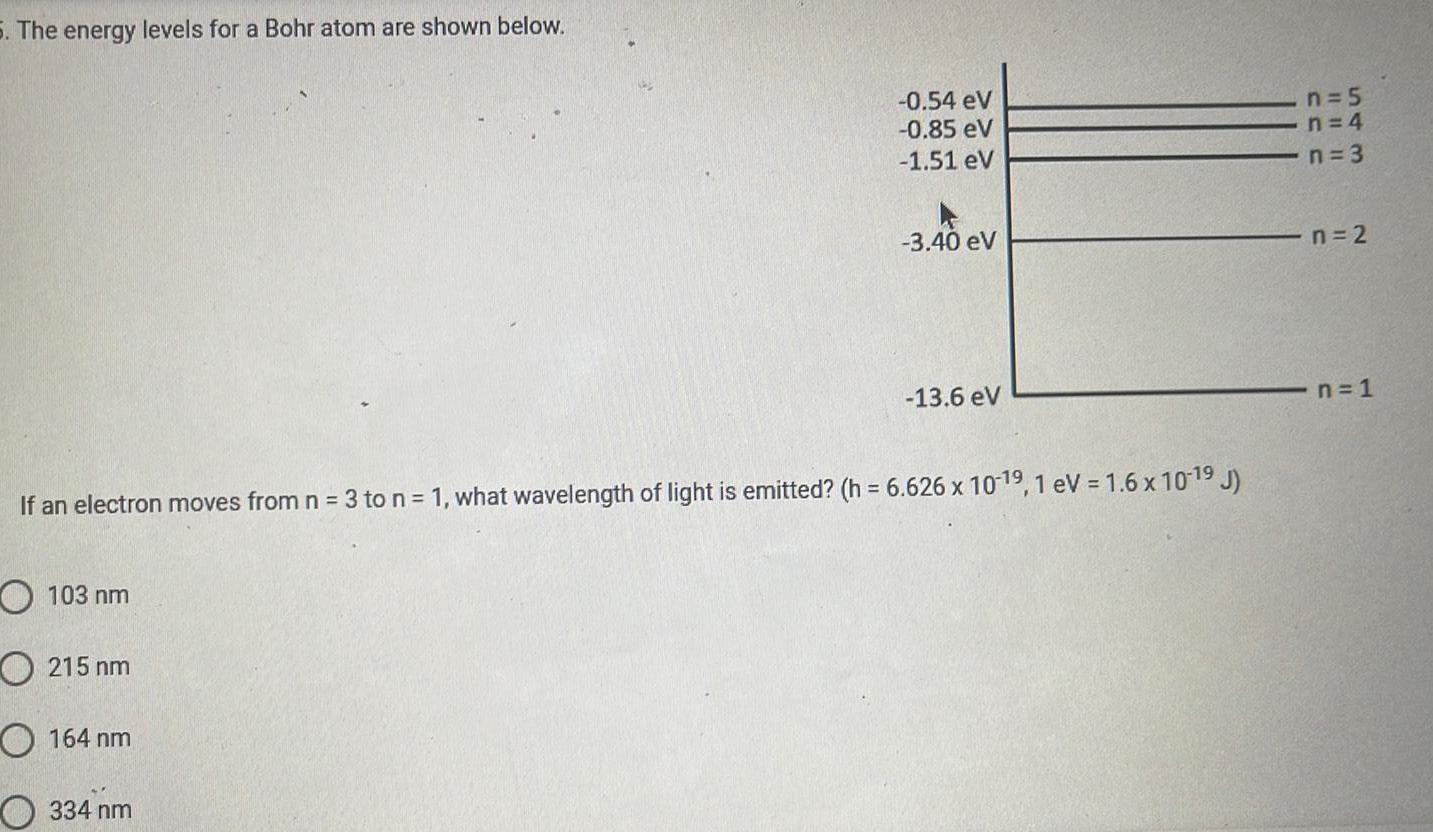Physics
Atomic Structure
The energy levels for a Bohr atom are shown below. If an electron moves from n = 3 to n = 1, what wavelength of light is emitted? (h= 6.626 x 10-19, 1 eV = 1.6 x 10-19 J) 103 nm 215 nm 164 nm 334 nm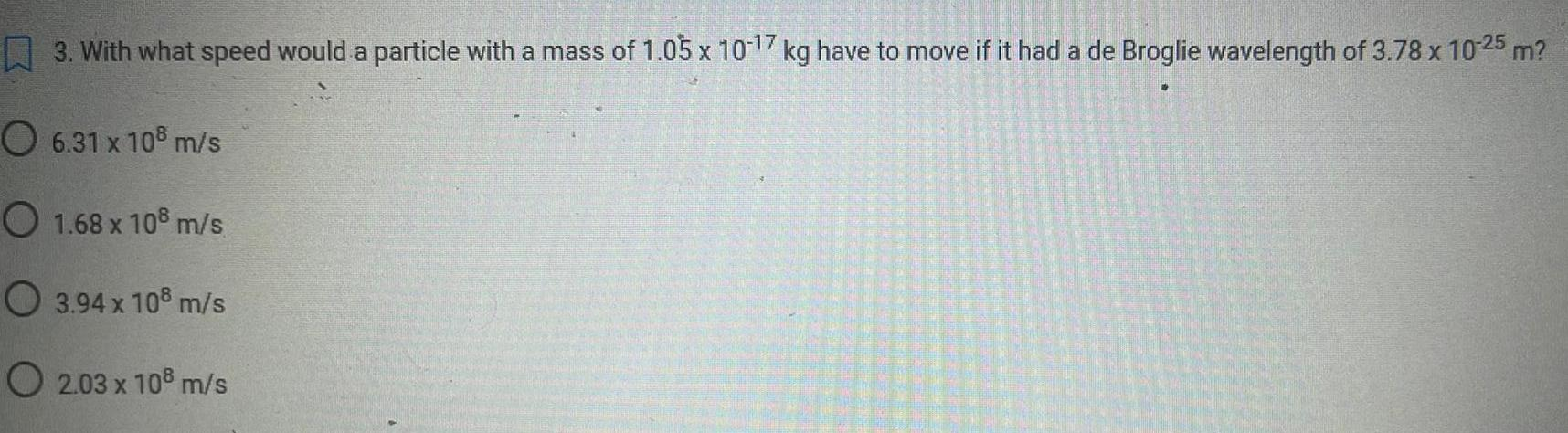Physics
Atomic Structure
3. With what speed would a particle with a mass of 1.05 x 10-17 kg have to move if it had a de Broglie wavelength of 3.78 x 10-25 m? 6.31 x 108 m/s 1.68 x 108 m/s 3.94 x 108 m/s 2.03 x 108 m/s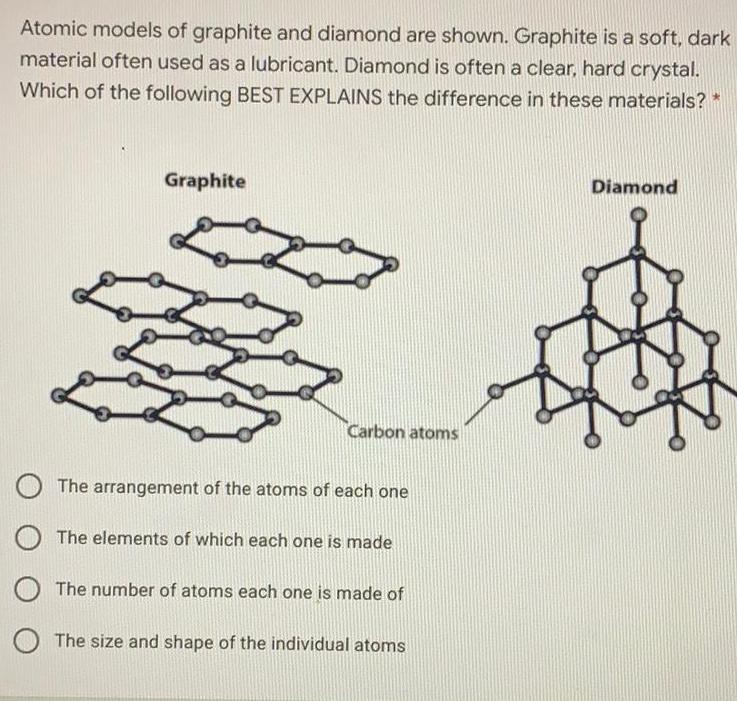Physics
Atomic Structure
Atomic models of graphite and diamond are shown. Graphite is a soft, dark material often used as a lubricant. Diamond is often a clear, hard crystal. Which of the following BEST EXPLAINS the difference in these materials? * The arrangement of the atoms of each one The elements of which each one is made The number of atoms each one is made of The size and shape of the individual atoms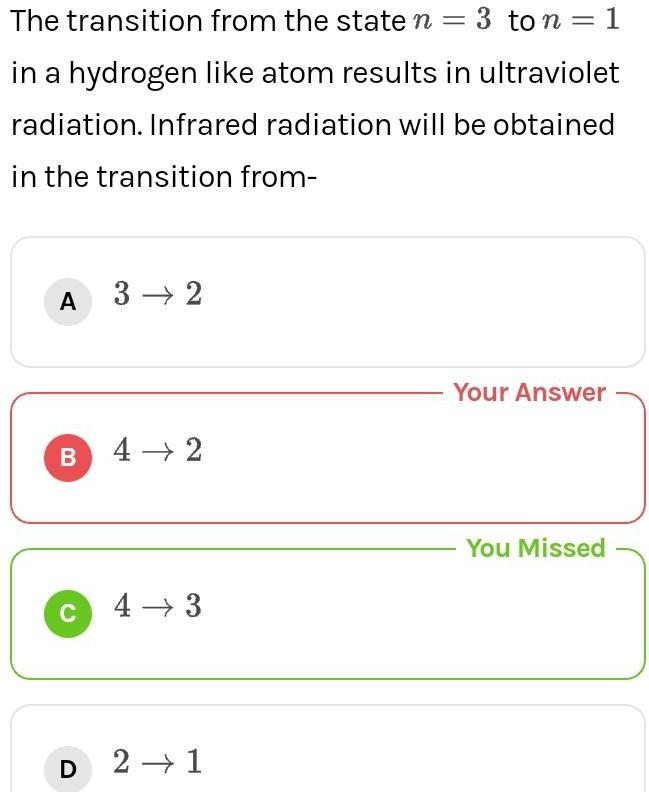Physics
Atomic Structure
The transition from the state n = 3 ton = 1 in a hydrogen like atom results in ultraviolet radiation. Infrared radiation will be obtained in the transition from- 3→ 2 4 → 2 4 → 3 2 →1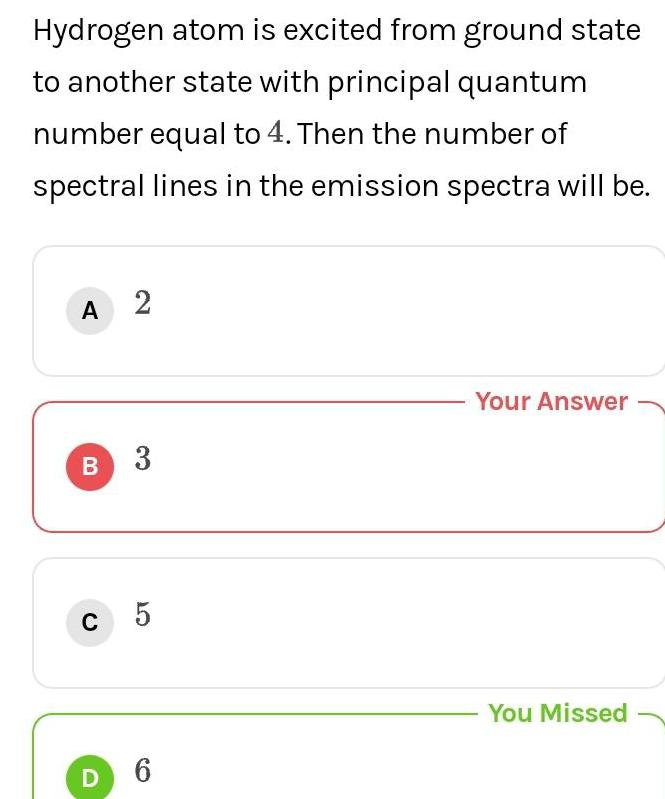Physics
Atomic Structure
Hydrogen atom is excited from ground state to another state with principal quantum number equal to 4. Then the number of spectral lines in the emission spectra will be. A 2 B 3 C 5 D 6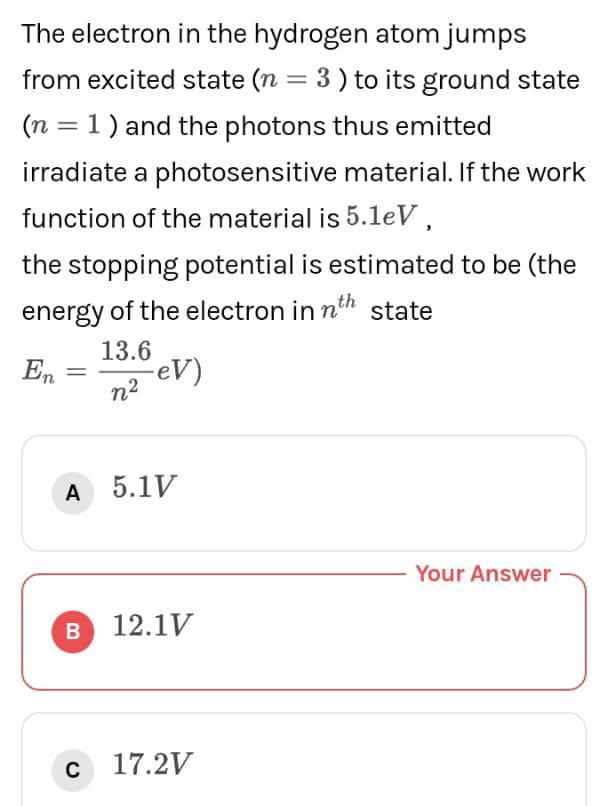Physics
Atomic Structure
The electron in the hydrogen atom jumps from excited state (n = 3) to its ground state (n = 1) and the photons thus emitted irradiate a photosensitive material. If the work function of the material is 5.1eV, the stopping potential is estimated to be (the energy of the electron in nth state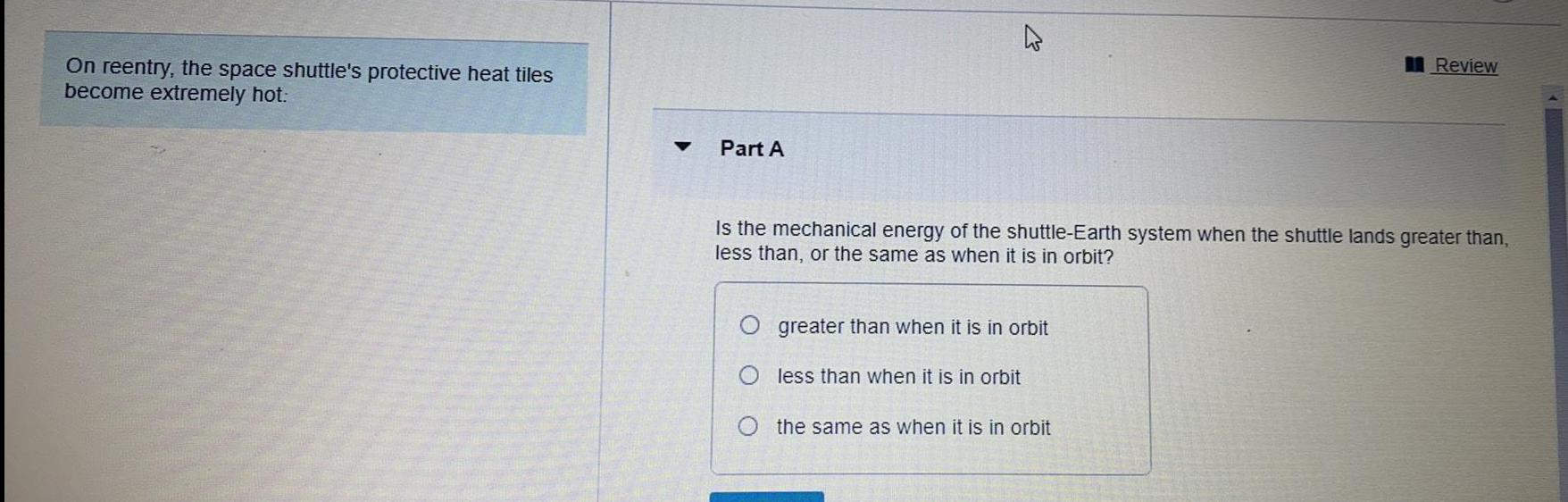Physics
Atomic Structure
On reentry, the space shuttle's protective heat tiles become extremely hot: Part A Is the mechanical energy of the shuttle-Earth system when the shuttle lands greater than, less than, or the same as when it is in orbit? greater than when it is in orbit less than when it is in orbit the same as when it is in orbit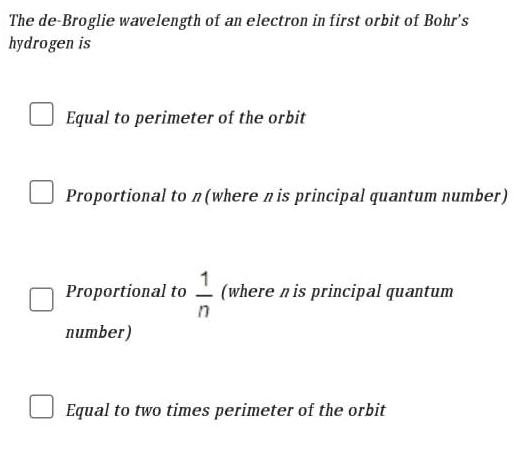Physics
Atomic Structure
The de-Broglie wavelength of an electron in first orbit of Bohr's hydrogen is Equal to perimeter of the orbit Proportional to n (where n is principal quantum number) Proportional to 1/n (where nis principal quantum number) Equal to two times perimeter of the orbit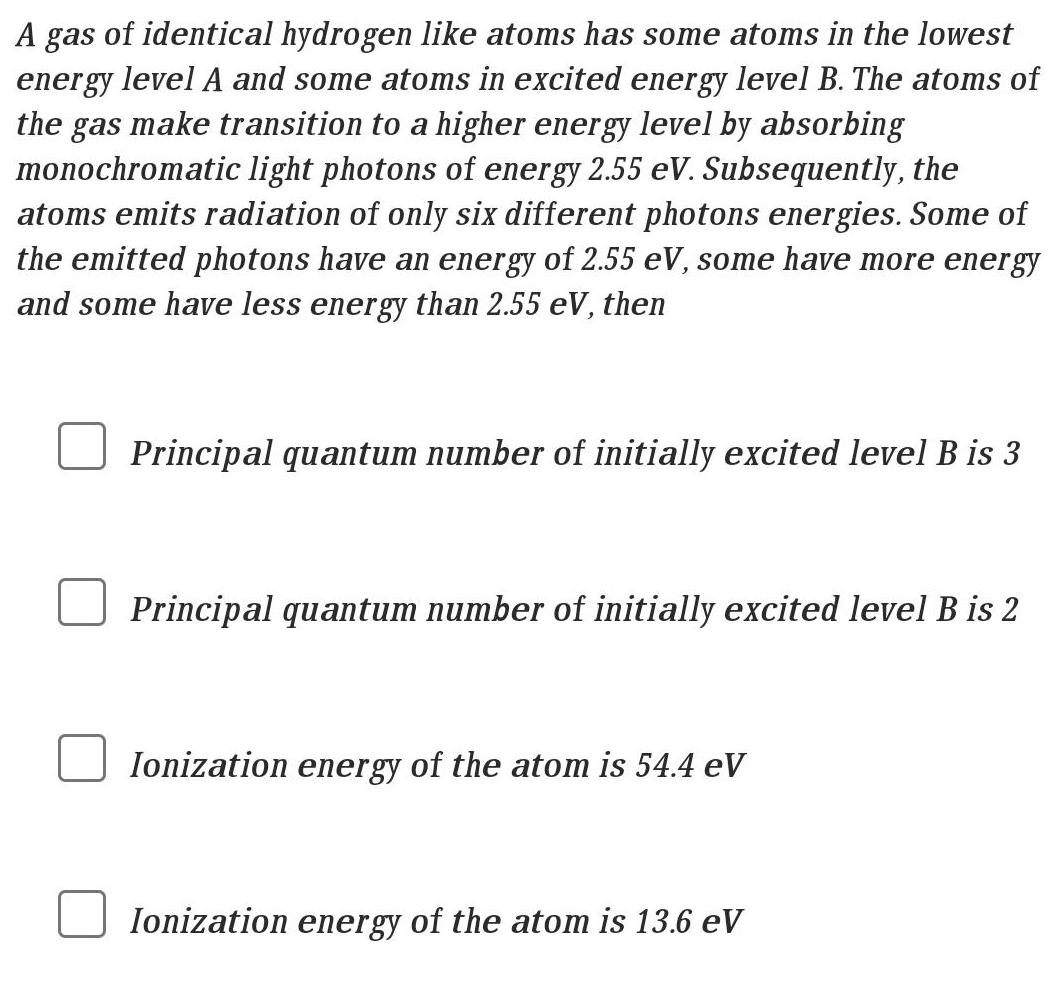Physics
Atomic Structure
A gas of identical hydrogen like atoms has some atoms in the lowest energy level A and some atoms in excited energy level B. The atoms of the gas make transition to a higher energy level by absorbing monochromatic light photons of energy 2.55 eV. Subsequently, the atoms emits radiation of only six different photons energies. Some of the emitted photons have an energy of 2.55 eV, some have more energy and some have less energy than 2.55 eV, then Principal quantum number of initially excited level B is 3 Principal quantum number of initially excited level B is 2 Ionization energy of the atom is 54.4 eV Ionization energy of the atom is 13.6 eV